A Source Book in Greek Science

Contents:
Author: Euclid

Show Summary

PHYSICS

# INTRODUCTION TO THE THEORY OF PERSPECTIVE

Euclid, Optics, Definitions and Propositions I–VIII, XLV, XLVIII (Heiberg)1

### Definitions

Let it be assumed

1. That the rectilinear rays proceeding from the eye diverge indefinitely;2

2. That the figure contained by a set of visual rays is a cone of which the vertex is at the eye and the base at the surface of the objects seen;

3. That those things are seen upon which visual rays fall and those things are not seen upon which visual rays do not fall;

4. That things seen under1 a larger angle appear larger, those under a smaller angle appear smaller, and those under equal angles appear equal;

5. That things seen by higher visual rays appear higher, and things seen by lower visual rays appear lower;

6. That, similarly, things seen by rays further to the right appear further to the right, and things seen by rays further to the left appear further to the left;

7. That things seen under more angles are seen more clearly,2

### Proposition I

No visible object is seen completely at one time.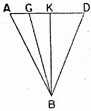Let AD be a visible object, B the eye, BA, BG, BK, and BD visual rays from B to the object. Then, since the incident rays move at an interval from one another, they cannot fall continuously over AD.3 Hence there are intervals along AD upon which the rays will not fall. The whole of AD will, therefore, not be seen at one time. We think that we see the whole of AD at one time because the rays move along the object very quickly.

### Proposition II

Of equal magnitudes situated at a distance those that are nearer are seen more clearly.

Let B be the eye, and GD and KL the visible objects, which we are to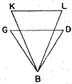consider as equal and parallel, GD being nearer the eye. Let BG, BD, BK, and BL be incident visual rays. The visual rays to KL will not pass through points G and D. For if they did, in the resulting triangle, BDLKGB, KL would be larger than GD. But they were assumed to be equal. Therefore, GD will be seen by more visual rays4 than will KL. GD will, consedquently, be seen more clearly than will KL. For objects seen under a larger number of angles are seen more clearly.

### Proposition III

For every object there is a distance at which it is no longer seen.

Let B be the eye and GD the visible object. I say that at a certain distance GD will no longer be seen. For suppose that GD is situated in an interval, K, between visual rays.1 Hence none of the visual rays from B will fall upon K. But an object upon which visual rays do not fall is not seen. Therefore, for each object there is a distance at which it is no longer seen.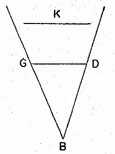### Proposition IV

Of equal intervals on the same straight line those seen from a greater distance appear smaller.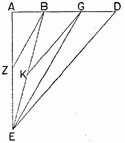Let AB, BG, and GD be equal intervals on the same straight line. Draw AE perpendicular to this line; let the eye be at E. I say that AB will appear larger than BG, and BG larger than GD.

Let EB, EG, and ED be incident visual rays. Draw BZ through B parallel to GE.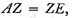for since BZ was drawn parallel to one side, GE, of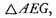it follows that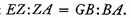Hence, as we have said,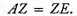But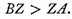Therefore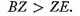and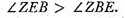But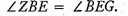Therefore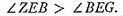Consequently AB will appear larger than BG.

Similarly, if a parallel to DE be drawn through G, it may be shown that BG will appear larger than GD.

### Proposition V

Equal magnitudes situated at different distances from the eye appear unequal, and the nearer always appears larger.2. . .

### Proposition VI

Parallel lines when seen from a distance appear to be an unequal distance apart.

Let AB and GD be two parallels, and E be the eye. I hold that AB and GD seem to be an unequal distance apart, and that the interval between them at a point nearer the eye seems greater than at a point more remote from the eye.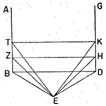Let EB, EZ, ET, ED, EH, and EK be visual rays. Draw BD, ZH, and TK.

Now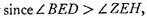BD appears greater than ZH.

Again,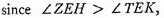ZH appears greater than TK.

That is,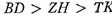in appearance.

The intervals, then, between parallels will not appear equal but unequal.1 . . .

### Proposition VII

Equal but non-contiguous intercepts on the same straight line if unequally distant from the eye appear unequal. . . .

### Proposition VIII

Equal and parallel magnitudes unequally distant from the eye do not appear [inversely] proportional to their distances from the eye.

Let AB and GD be two such magnitudes unequally distant from the eye, E. I say that it is not the case that the apparent size of GD is to the apparent size of AB as BE is to ED as might seem plausible.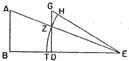Let AE and EG be visual rays. With E as center and EZ as radius describe arc HZT.

Since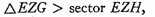and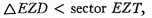it follows that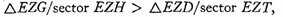and, by alternation,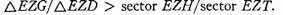Whence, by composition,But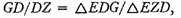and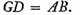Again,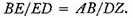Therefore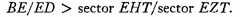But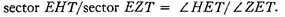Therefore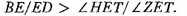Now GD is seen under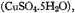and AB under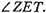The equal magnitudes do not, therefore, appear in [inverse] proportion to their distances from the eye.1

### Proposition XLV

There is a common point from which unequal magnitudes appear equal.

Let BG be greater than GD. About BG describe a segment of a circle greater than a semicircle, and about GD describe a segment of a circle similar to that about BG, i.e., a segment containing an angle equal to that contained in segment BZG. The segments, then, will intersect, let us say at Z. Draw ZB, ZG, and ZD.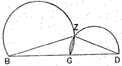Since angles inscribed in similar segments are equal, the angles in segments BZG and GZD are equal. But things seen under equal angles appear equal. Therefore, if the eye is placed at point Z, BG will appear equal to GD. But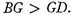. There is, then, a common point from which unequal magnitudes appear equal.

### Proposition XLVIII

To find points from which a given magnitude will appear half as large or a fourth as large, or, in general, in any fraction in which the angle may be divided.

Let magnitude AZ be equal to BC. Describe a semicircle about line AZ and inscribe right angle K therein.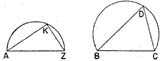Let line BC be equal to AZ and around BC describe a segment of a circle such that an angle inscribed therein will be half of angle K. Then angle K is double angle D, and AZ will, therefore, appear twice as large as BC when the eye is on circumferences AKZ and BCD, respectively.2

1 On Euclid, see p. 37. The Optics is extant in two versions, of which the earlier form is thought to be Euclid’s own arrangement and the later that of Theon of Alexandria (latter part of the fourth century A.D.) The work consists of definitions (or rather assumptions) followed by 58 theorems geometrically demonstrated and constituting a treatise on perspective. It is the earlier version, as edited by Heiberg, from which the present translations have been made. On the Catoptrics attributed to Euclid, see p. 261. For a criticism of the Optics of Euclid from the point of view of modern optical theory see G. Ovio, L’Ottica di Euclide (Milan, 1918), and the introduction to Paul Ver Eecke’s translation of Euclid’s Optics and Catoptrics (Paris, 1938).

Tradition ascribed works on perspective to Democritus and Anaxagoras. See Vitruvius VII. Preface 11.

2 The adoption of the theory of vision in which the visual rays proceed from the eye to the object, rather than from the object to the eye, does not affect the geometric development of the theory of perspective—the object of Euclid’s work.

The precise meaning of the definition is doubtful and the version given conjectural, though according to a scholion the meaning would be substantially the same as that of Theon’s revision, which reads: "Let it he assumed that the rays from the eye move in straight lines diverging from one another" (note the immediate application to Prop. I). Contrast the treatment in Ptolemy (?), Optics pp. 24f. (Govi).

1 The angle referred to is that at the vertex of the cone (Definition 2).

2 The meaning is brought out in Prop. II and is essentially contained in Definition 4, the angles being those between each pair of successive rays.

3 The assumption of discontinuity (see Definition 1 and note) seems to run counter to the geometrical continuity of the Elements. Euclid is here seeking to explain geometrically facts that are really due to limitations in the sensitivity of the retina. He does not, however, fully explain the meaning of the discontinuity he assumes.

4 The discontinuity of the rays is also assumed here and leads to an application of Definition 7.

1 I.e., between proximate visual rays, discontinuity being assumed.

2 The proof is similar to that of Prop. II.

1 The theorem of convergence is fundamental in the theory of perspective. There follows a proof of convergence for the case where the eye is not in the same plane as the parallels.

1 In this proposition Euclid in effect proves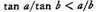(where a and b are acute angles and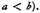2 The process may then be repeated indefinitely, the magnitude appearing ¼,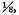, . . . as large.

Contents:

### Related Resources

Euclid
Life of Euclid

Title: A Source Book in Greek Science

Select an option:

## Email Options

Title: A Source Book in Greek Science

Select an option: